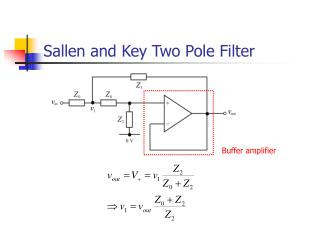DownloadDownload PresentationSallen and Key Two Pole Filter

# Sallen and Key Two Pole Filter

Télécharger la présentation## Sallen and Key Two Pole Filter

- - - - - - - - - - - - - - - - - - - - - - - - - - - E N D - - - - - - - - - - - - - - - - - - - - - - - - - - -
##### Presentation Transcript

1. Buffer amplifier Sallen and Key Two Pole Filter

2. But Apply Kirchoff’s current law to v1 node:

3. Substituting,

4. Two-Pole Low-Pass Filter

5. Two-Pole High-Pass Filter

6. So, Eg. Two-Pole Example Design a second order normalised Butterworth low pass filter.

7. Higher Order Filters • Using a single op-amp the highest order practical filter design is two. • For higher order filters, more than one op-amp is needed. • Simplest design technique is cascade synthesis or synthesis by sections.

8. Synthesis by Sections • When two (or more) filters are cascaded in series, the total transfer function is the product of the individual transfer functions. • HTOT(s) = H1(s) H2(s) • The required transfer function must be factorised into second order sections. H1(s) H2(s) VIN(s) VIN(s)H1(s) VIN(s)H1(s) H2(s)

9. Factorising the Transfer Function • Filter design techniques usually generate the positions of the poles (and zeros) of the transfer function. • Grouping complex conjugate pairs together is essential for physically realisable sections. • If the required filter order is odd, a single pole will be left over. This is realised by a first order section.

10. Transfer function is, therefore: Fourth Order Example The poles of a normalised fourth order low pass Butterworth filter are:

11. Fourth Order Realisation

12. Third Order Example The poles of a normalised third order low pass Bessel filter are: Transfer function is, therefore:

13. Ordering the Sections • In theory, using ideal op-amps, it doesn’t matter which section is first or second. • In practice, the decision is based on considerations of: • Saturation Levels • Noise Figure From fourth order example:

14. Second Order Frequency Response • If the damping ratio, z, is less than 1/Ö2, the peak gain is greater than one. • This means that the maximum input level is reduced, to avoid op-amp saturation. 10 z=0.2 0.707 1 0.5 1 Gain z=2 0.1 0.01 1 0.1 10 Frequency [rad/s]

15. Butterworth Filter Example

16. H1(s) H2(s) H2(s) H1(s) At w = 1 rad/s,

17. Section Ordering • To get the highest maximum input signal range. • Sections with the largest damping ratio (lowest Q) should come first. • Resonant sections with low damping ratios come last, possibly susceptible to noise. • To get the lowest output noise. • Highest gain sections (i.e. most resonant) should come first. • Subsequent sections attenuate noise. • What’s the best order ? • It depends.

18. Summary • The Sallen and Key configuration realises a two-pole filter section using a single op-amp. • Synthesis by sections creates higher order filters by cascading first and second order sections. • Under ideal assumptions, the order of the sections is irrelevant. • Practically, dynamic range considerations decide the order of the sections.